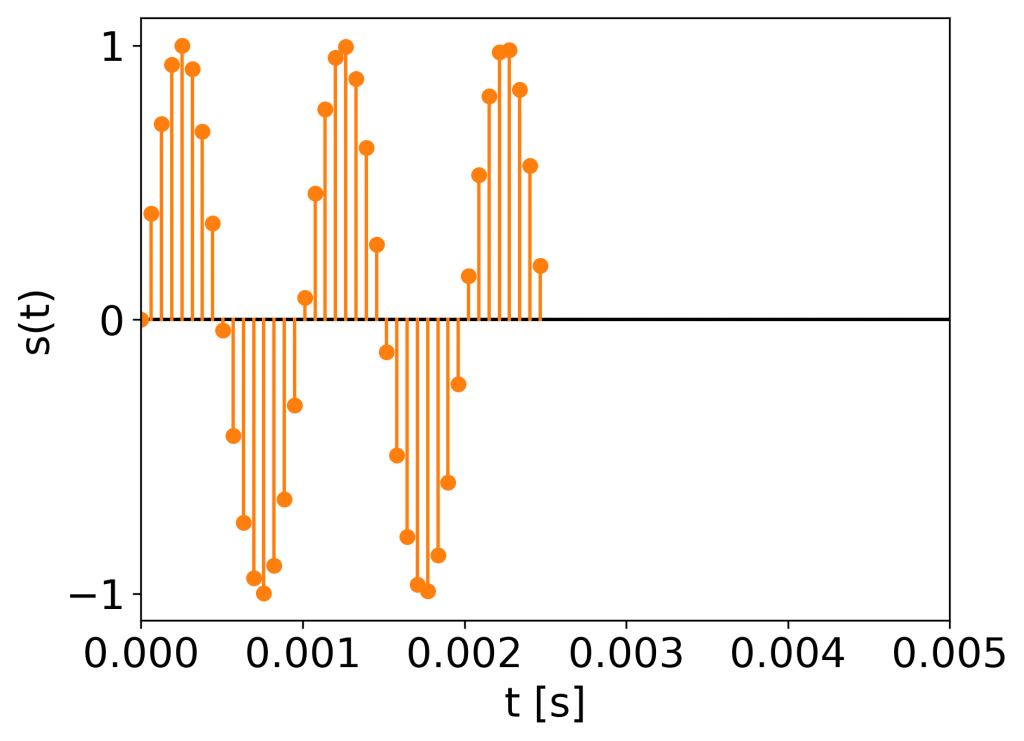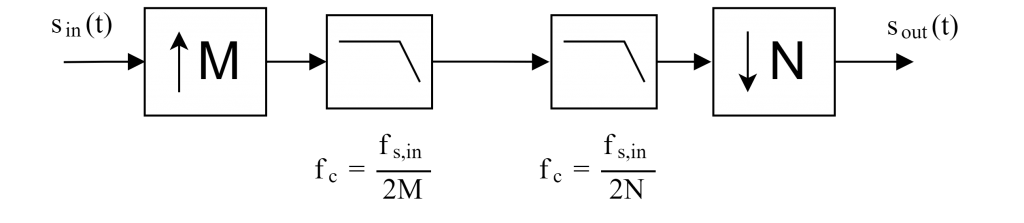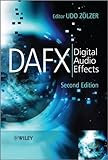Do you remember the slowdown of time in “Inception”? Or the “bullet time” in “The Matrix”? How to create such a slow motion effect in the audio domain? That is the topic of today’s article!

In this article we will prove the usefulness of the sampling theory in music and audio effects. If you don’t know what sampling is all about check out this article. If you need a revision on aliasing check out this article.

## Decimation in time

What would happen if we removed every other sample from the signal? To simplify things, let’s look at an example.

Here we have a 500 Hz sine wave sampled at 16 kHz:500 Hz sine sampled at 16,000 Hz.

Let’s note, that one period is 2 ms long.

Removing every second sample we obtain:The signal after removing every other sample.

We can see, that the duration of the sine shrunk to 2.5 ms, what is no surprise, since we basically removed half of the signal and 2.5 ms corresponds to half of 5 ms.

But if duration changed, with sample rate remaining constant, the frequency changed as well! The new period length is 1 ms, so the frequency of the sine is not 500 Hz anymore, but $\frac{1}{0.001} = 1000$ Hz!

The process of removing particular samples is called decimation or downsampling and here we performed decimation with decimation factor equal to 2 (2 times less samples). It sometimes involves the antialiasing filter, which will be explained below in more detail.

## Tape replay

If you ever rewinded a cassette magnetic tape, you were able to hear a quickened version of the recording, what enabled you to find the exact spot you wanted to hear. That’s exactly the same effect you can witness by sample decimation.

We can view that change in the signal as though the replay sample rate has changed. If we output the samples with two times the original sample rate we will run out of samples in half of the duration of the original signal, since we are outputting twice as many samples per second as before.

### Mathematical formulation

We may express it in mathematical terms as the length of the output signal’s period in seconds:

$T_{replay} = \frac{T_{in}}{v}, \text{ \{eq:vsr\_time\}}$

where $T_{replay}$ is the time period of the output signal, $T_{in}$ is the time period of the original signal and $v$ is the relative speed of the “tape” (i.e., how many times quicker we output the sound) compared to the original or default. For instance, playing the sound two times faster ($v = 2$) produces a two times shorter waveform at the output (of time period length equal to $\frac{T_{in}}{2}$ seconds).

Converting to the frequency domain means taking the reciprocal of equation {eq:vsr_time} what results in the following frequency scaling:

$f_{replay} = f_{in} v,$

where $f_{replay}$ denotes the frequency of the output signal given the frequency of the input signal $f_{in}$ and the scaling (replay speed) $v$. This result is very descriptive, because it basically says, that the frequency of the transformed signal will be scaled along with $v$.

### Possible ways to rescale signal’s time

How can we achieve the effect of variable speed replay using digital devices rather than a tape player? There are basically two ways:

• change the sampling frequency of the DAC (e.g., in a stage setting),
• resample the signal before output.

As this is a blog about audio programming we will deal with the second option, namely the variable speed replay algorithm.

## Variable speed replay algorithm

Assuming that $v$ can be displayed as a ratio of integer numbers $v = \frac{N}{M}$, the schema of the variable speed replay algorithm is as follows:Variable speed replay algorithm scheme.

Here $s_{in}(t)$ and $s_{out}(t)$ denote discrete-time input and output signals respectively, $f_c$ the cutoff frequency of the low-pass filter, $f_{s, in}$ sample rate of the input signal, and $M$ and $N$ are integers such that $v=\frac{N}{M}$. The following subsequent operations are applied to the input signal:

• upsampling by a factor of M (inserting M-1 zeros after each sample),
• low-pass filtering with cutoff frequency set to $\frac{f_{s, in}}{2M}$ (to remove the repeated spectral components that were dragged below the Nyquist frequency as a result of lowering the frequency of the repeated digital spectra),
• low-pass filtering with cutoff frequency set to $\frac{f_{s, in}}{2N}$ (to prevent the aliasing of the stretched spectra, since all repeated spectral components will be stretched by downsampling),
• downsampling by a factor of N (removing N-1 samples from each block of N samples, exactly what we did to our 500 Hz sine).

Note the usage of low-pass filters. They are crucial to prevent any additional components from appearing in the processed signal’s spectrum. So whether we are raising the frequency or we are lowering it, we must always filter, to remove the extra components interfering with our original signal.

Upsampling is performed first, because downsampling (removing certain samples) may be considered a removal of information, therefore it’s done best at the end of processing.

All in all, this scheme is a typical resampling scheme. The only difference in the variable speed replay algorithm is that we treat the output signal, as if it still had the original sample rate $f_{s, in}$ and thus the difference in pitch when playing it out.

## The outcome of variable speed replay application

We can reason about the result of such signal rescaling in two domains: time and frequency.

### Modified frequency structure

The frequency structure changes with the scaling accordingly:

• if we are quickening the signal (scaling the time speed up) we receive a “Mickey Mouse” or a “Chipmunk” effect (especially powerful when applied to speech),
• if we are slowing the signal (scaling the time speed down) we perceive a “slow motion” effect (exactly like the one in “Inception” or “The Matrix”)

### Modified time structure

The time structure is altered with the following perceivable changes:

• the transients are spread (slower) or contracted (more rapid),
• the vibrato technique (changing the frequency of the instrument in a certain range around the played note) loses its characteristics: it becomes a slower (when slowing down time) or faster modulation.

### Negative speed?!

If we set $v=-1$ we get… a time-reversed signal! This technique is extremely powerful, especially in experimental or progressive music, e.g. solo on “Misunderstood” by Dream Theater has been time-reversed (check out the whole story on Wikipedia) or ending of “A Day in the Life” by The Beatles, where one can hear reversed recordings being played in a loop.

Keeping the $v$ ‘s value negative we can change the scaling of the inversed time signal… What gives an enormous amount of possibilities! Check out this example in Ludger Brümmer’s “The Gates Of H” musique concreté piece.

Above examples show that variable speed replay algorithm is a creative application of the sampling theory.

## Unity Time.timeScale correspondence

If you ever worked with the Unity Editor, you may have stumbled upon the Time.timeScale parameter. It basically changes the speed at which things are happening. If set to 1 the time flows normally, however, if you decrease it to 0.5 the events happen two times slower, so the Time.timeScale parameter corresponds to the $v$ parameter in the variable speed replay algorithm. It doesn’t change the pitch and time duration of audio though, but… you could do that using the algorithm described here! Actually that’s what the pitch parameter of AudioSource component does: it changes pitch and time simultaneously, taking advantage of the Variable Speed Replay algorithm. Read the corresponding Unity manual parts here for the timeScale parameter and here for the pitch parameter.

Note: the Time.timeScale parameter also does not change the rate at which physics engine is updating. Thus the corresponding Time.fixedDeltaTime parameter should be updated accordingly (typically Time.fixedDeltaTime = 0.02f * Time.timeScale, where 0.02f denotes the typical default value of Time.fixedDeltaTime, but it can be different of course).

## Time scaling preserving pitch

It is not trivial to scale the signal in time without altering the pitch (i.e. to lengthen or shorten the signal). Here, a variety of Overlap-Add methods apply, which are beyond the scope of this article. However, they are likely to appear in the future WolfSound’s articles, so stay tuned!

## Summary

We have discussed the variable speed replay algorithm, which enables to manipulate the relative feeling of time flow in the signal and explored its consequences and applications.

If you want to learn more I highly encourage you to check out this book “Digital Audio FX” by Udo Zölzer et. al.:It contains an even broader perspective on the variable speed replay algorithm and many more; it is one of the best books to learn about audio algorithms!

## Code example

The following code is a command-line tool to create the slow-motion or ‘chipmunk’ sound effect. The ‘crude’ version is a direct implementation of the algorithm as presented on the scheme above, the ‘smart’ version uses the resampling facility of SciPy and is significantly faster.

#!/usr/bin/env python3
"""
Variable speed replay algorithm implementation.

A command-line tool that processes the supplied wave file and plays out the result.
"""
import argparse
from scipy.signal import resample, butter, lfilter
import sounddevice as sd
import numpy as np
__author__  = "Jan Wilczek"
__version__ = "1.0.0"

def parse_args():
parser = argparse.ArgumentParser(description='Apply the Variable Speed Replay algorithm to an audio file '
'and play it out.')
parser.add_argument('-f', '--filename', type=str, required=True, help='filename of the wave file to process',
dest='filename')
parser.add_argument('-N', type=int, required=True, help='numerator of the time scale parameter (v = N / M)',
dest='N')
parser.add_argument('-M', type=int, required=True, help='denominator of the time scale parameter (v = N / M)',
dest='M')
default=process_frame_smart,
help='process each frame with brute force implementation '
'(default: process each frame optimally)')
return parser.parse_args()

data = data[:, 0]                           # extract first channel
normalized_data = data / np.amax(abs(data)) # convert to [-1, 1] range
return sample_rate, normalized_data

def play_samples_blocking(samples, sample_rate, volume=1.0):
sd.play(volume * samples, samplerate=sample_rate)
sd.wait()

def upsample(frame, M):
M_minus_1_zeros = (M - 1) * [0.0]
for i in range(1, len(frame)):
next_sample_id = (i-1) * M + 1
frame = np.insert(frame, next_sample_id, M_minus_1_zeros)
return frame

def filter_frame(frame, nyquist_frequency, M, N):
order = 5
cutoff_frequency = min(nyquist_frequency / M, nyquist_frequency / N)
normalized_cutoff = cutoff_frequency / nyquist_frequency
b, a = butter(order, normalized_cutoff, btype='low', analog=False)
return lfilter(b, a, frame)

def downsample(frame, N):
return [frame[i] for i in range(0, len(frame)) if i % N == 0]

def process_frame_crude(frame, M, N, fs):
upsampled = upsample(frame, M)
filtered = filter_frame(upsampled, fs / 2, M, N)
downsampled = downsample(filtered, N)
return downsampled

def process_frame_smart(frame, M, N, _):
return resample(frame, int(len(frame) / (N / M)))

def variable_speed_replay(samples, fs, M, N, process_frame_function, frame_size=16384):
if N / M == 1:
return samples

output = np.empty((0,))
for i in range(0, len(samples), frame_size):
end = min(i + frame_size, len(samples))
resampled_frame = process_frame_function(samples[i:end], M, N, fs)
output = np.append(output, resampled_frame)
return output

if __name__ == '__main__':
args = parse_args()


Feel free to copy and test out the above code. If you are not sure how to use it, then run variable_speed_replay.py --help (assuming you copied the code into ‘variable_speed_replay.py’ file) and follow the instructions given.